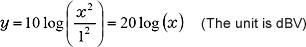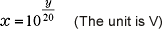FFT analyzer FAQWhat does the dB value of the power spectrum vertical axis stands for?The dB (decibel) is defined as 10 times of the logarithm to the ratio of the reference value of selected physical amount. Generally, the dB value is a relative value, but the unit for the power spectrum dB value before the physical calibration is V2, so the dBV which sets 1 V2 (the square of rms) as a reference value is displayed as the absolute unit. According to this, if the power spectrum of the selected frequency is x2, the dB value can be calculated in the following expression (1);(1)

Conversely, if the power spectrum value is y (dBV), the linear spectrum x can be calculated in the following expression (2);(2)

Please refer to the example values of the linear spectrum x (V) and power spectrum y (dBV) in the following diagram 1.

Diagram 1: The specific example value of the power spectrum and linear spectrum

Power spectrum(V2) Power spectrum(dBV) Linear spectrum(V)
0.0001 -40 0.01
0.01 -20 0.1
0.5 -3.01 0.707
10 10 3.16
100 20 10

The power spectrum is a square average value (effective value), so sometimes the unit "dBVr" is displayed to make it more understandable.

Revised:2009/11/16